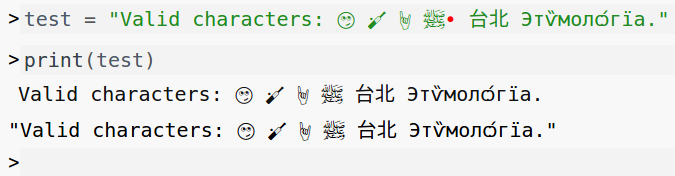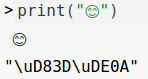#### 3.3Strings

The type of string values.

A String is a fixed-length array of characters. This includes not only letters in the Latin alphabet and numerals, but any Unicode character, including languages using non-Latin characters, such as Arabic, Russian or Chinese, as well as emoji defined in the Unicode specification.If you click on printed strings in the interactive window, the display will toggle between the character itself and the relevant Unicode escape code or codes.Internally, a Pyret String is implemented as a JavaScript JSString. See the Runtime API for more context.

One implication of how JavaScript handles Unicode characters is that characters that are identified by a Unicode code point greater than 65535 are sometimes treated as two characters by Pyret, as noted below.

##### 3.3.1String Functions

string-equal :: (s1 :: String, s2 :: String) -> Boolean

Returns true if the two strings are equal.

Examples:
```check:
string-equal("abc", "abc") is true
"abc" is%(string-equal) "abc"
"abc" == "abc" is true
string-equal("ab", "abc") is false
string-equal("abc     ", "abc") is false
end```

string-contains :: (string-to-search :: String, string-to-find :: String) -> Boolean

Returns true if string-to-find is contained in string-to-search. Returns true if an empty string is passed as string-to-find.

Examples:
```check:
string-contains("Ahoy, world!", "world") is true
string-contains("Ahoy, World!", "world") is false
string-contains("world", "Ahoy world") is false
string-contains("same string", "same string") is true
string-contains("", "") is true
string-contains("any string", "") is true
end```

string-append :: (front :: String, back :: String) -> String

Returns a String where back is added to the right of front.

Examples:
```check:
string-append("a", "b") is "ab"
string-append("same", "same") is "samesame"
string-append("", "") is ""
string-append("", "a") is "a"
string-append("a", "") is "a"
end```

Returns the number of characters in the string.

string-length reports a count of 2 for code points over 65535.

Examples:
```check:
string-length("") is 0
string-length("    ") is 4
string-length("four") is 4
string-length("🏏") is 2
end```

Converts the argument string to a number, returning none if it is not a valid numeric string, and some number if it is.

string-to-number is strict about its inputs, and recognizes exactly the same numbers that Pyret itself does: no surrounding whitespace, extra punctuation, or trailing characters are allowed.

Examples:
```check:
string-to-number("100") is some(100)
string-to-number("not-a-number") is none
string-to-number(" 100") is none
string-to-number("100abc") is none
string-to-number("1,000") is none
string-to-number("1-800-555-1212") is none
end```

string-repeat :: (s :: String, n :: Number) -> String

Examples:
```check:
string-repeat("a", 5) is "aaaaa"
string-repeat("", 1000000) is ""
string-repeat("word ", 3) is "word word word "
string-repeat("long string", 0) is ""
end```

string-substring :: (
s :: String,
start-index :: Number,
end-index :: Number
)
-> String

Returns a new string created from the characters of the input string, starting from start-index (inclusive) and ending at end-index (exclusive). Raises an exception if start-index is greater than end-index, if start-index is greater than the length of the string, or if end-index is less than 0.

The returned string always has length end-index - start-index.

String indexes are counted starting from zero for the first character.

Examples:
```check:
string-substring("just the first", 0, 1) is "j"
string-substring("same index", 4, 4) is ""

tws = "length is 12"
string-substring(tws, 4, 6) is "th"
string-substring(tws, string-length(tws) - 1, string-length(tws)) is "2"
string-substring(tws, 6, 4) raises "index"
string-substring(tws, 6, 13) raises "index"
string-substring(tws, 13, 6) raises "index"
string-substring(tws, -1, 10) raises "index"
end```

string-index-of :: (original-string :: String, string-to-find :: String) -> Number

Returns the index from the beginning of the string where string-to-find first appears, or -1 if the string isn’t found.

Examples:
```check:
string-index-of("Pyret", "P") is 0
string-index-of("012🤑45", "🤑") is 3
string-index-of("🤔🤔🤔", "🤒") is -1
end```

string-replace :: (
original-string :: String,
string-to-find :: String,
replacement-string :: String
)
-> String

Returns a string where each instance of string-to-find in the original-string is replaced by replacement-string.

If the string to find is empty "", the replacement-string will be added between characters but not at the beginning or end of the string.

Examples:
```check:
string-replace("spaces to hyphens", " ", "-") is "spaces-to-hyphens"
string-replace("remove: the: colons", ":", "") is "remove the colons"
string-replace("😊😊🤕😊", "🤕", "😊") is "😊😊😊😊"
string-replace("rinky dinky", "inky", "azzle") is "razzle dazzle"
string-replace("a string", "not found", "not replaced") is "a string"
string-replace("", "", "c") is ""
string-replace("aaa", "", "b") is "ababa"
end```

string-split :: (original-string :: String, string-to-split-on :: String) -> List<String>

Searches for string-to-split-on in original-string. If it is not found, returns a List containing original-string as its single element.

If it is found, it returns a two-element List, whose first element is the portion of the string before first occurence of string-to-split-on. The second element contains the portion of the string after. The string-to-split-on is not included in either string. The string before and the string after might be empty.

For splitting beyond the first occurence of the string, see string-split-all.

Examples:
```check:
string-split("string", "g") is [list: "strin", ""]
string-split("string", "") is [list: "", "string"]
string-split("a-b-c", "-") is [list: "a", "b-c"]
end```

string-split-all :: (original-string :: String, string-to-split-on :: String) -> List<String>

Searches for string-to-split-on in original-string. If it is not found, returns a List containing original-string as its single element.

If it is found, it returns a List, whose elements are the portions of the string that appear in between occurences of string-to-split-on. A match at the beginning or end of the string will add an empty string to the beginning or end of the list, respectively. The empty string matches in between every pair of characters.

Examples:
```check:
string-split-all("a-b-c", "-") is [list: "a", "b", "c"]
string-split-all("split on spaces", " ") is [list: "split", "on", "spaces"]
string-split-all("explode", "") is [list: "e", "x", "p", "l", "o", "d", "e"]
string-split-all("bananarama", "na") is [list: "ba", "", "rama"]
string-split-all("bananarama", "a") is [list: "b", "n", "n", "r", "m", ""]
end```

A shorthand for string-split-all(s, "").

string-char-at :: (s :: String, n :: Number) -> String

Returns a String containing the character at the string index n from String n.

Examples:
```check:
string-char-at("abc", 1) is "b"
string-char-at("a", 0) is "a"
end```

Pyret uses JavaScript’s built-in string operations, and so will have the same behavior as toUpperCase.

Convert a string to all uppercase characters. Punctuation and other characters without an uppercase equivalent are left alone. Note that because of characters like ß, the length of the input is not guaranteed to match the length of the output.

Examples:
```check:
string-to-upper("a") is "A"
string-to-upper("I'm not yelling!") is "I'M NOT YELLING!"
string-to-upper("ß") is "SS"
string-to-upper("λαμβδα") is "ΛΑΜΒΔΑ"
string-to-upper("😊") is "😊"
string-to-upper(" ﷵ‎") is " ﷵ‎"
end```

When performing case-insensitive comparisons, it can be useful to convert both strings to uppercase first:

Examples:
```check:
string-to-upper("E.E. Cummings") is string-to-upper("e.e. cummings")
end```

Converts a String to all lower case.

Examples:
```check:
string-to-lower("A") is "a"
string-to-lower("I'M NOT YELLING!") is "i'm not yelling!"
string-to-lower("SS") is "ss"
string-to-lower("ΛΑΜΒΔΑ") is "λαμβδα"
end```

For strings that contain a single character whose code point is greater than 65535, this function raises an error. To get multiple codes at once for a longer string (or a string with larger code points), use string-to-code-points.

Converts s, which must be a single-character String, to a character code – a Number corresponding to its Unicode code point (http://en.wikipedia.org/wiki/Code_point).

Examples:
```check:
string-to-code-point("a") is 97
string-to-code-point("\n") is 10
string-to-code-point("λ") is 955
end```

Converts the string (of any length) to a list of code points. Note that strings are encoded in such a way that some characters correspond to two code points (see the note in string-to-code-point).

Examples:
```check:
string-to-code-points("") is [list:]
string-to-code-points("abc") is [list: 97, 98, 99]
string-to-code-points("😊") is [list: 55357, 56842]
string-to-code-points("𝄞") is [list: 55348, 56606]
end```

Code points greater than 65535 are not supported. You must encode higher code points with a surrogate pair in combination with string-from-code-points and string-to-code-points.

Converts the code point code to a Pyret string.

Examples:
```check:
string-from-code-point(97) is "a"
string-from-code-point(10) is "\n"
string-from-code-point(955) is "λ"
end```

Converts from a list of code points to a Pyret string.

Examples:
```check:
string-from-code-points([list:]) is ""
string-from-code-points([list: 97, 98, 99]) is "abc"
string-from-code-points([list: 55348, 56606]) is "𝄞"
end```